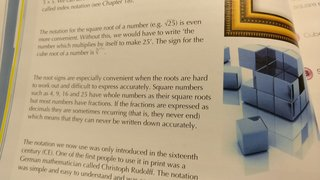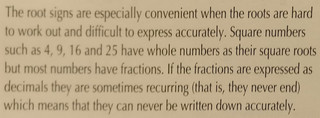# The Book Is Not Always Right

 Recent changes Table of contents
 Links to this page FRONT PAGE / INDEXSubscribe!My latest posts can be found here:
Previous blog posts:
Additionally, some earlier writings:## The book is not always right

Recently someone tweeted a picture of a page from a book. It seems to be some sort a book about maths aimed at students, but in one simple paragraph there was just so much wrong, it attracted many comments from many of my friends and colleagues.

But then someone I follow on twitter asked why it was so bad, and could it be explained to someone with no more than A-Level maths, and so I thought I'd give that a go. Here is my explanation.

Before I start, here's a comment prompted by one of my readers.

My non-mathematical reader might look at all of this and think of it as unimportant pedantry. But I think it's important to hear this message. The sense sometimes is that something might be technically wrong, but "close enough for Government work," but that view can be damaging and have life-long effects. The skills to think deeply, clearly, carefully, accurately, and precisely about things is becoming more and more important, and those skills are exactly those honed by doing mathematics.

So many "proofs" are wrong because someone didn't consider a negative square root, or didn't think hard enough about whether something was a rational or an irrational. If you don't form and reinforce the intuition that negatives are square roots, or that fractions and irrationals are very much not the same thing, you generate mathematicians whose instincts go against their own work.

Not everyone will be a mathematician, agreed, but the skills are eminently transferrable.

Here is the original text:

 "Square numbers such as 4, 9, 16, and 25, have whole numbers as their square roots, but most numbers have fractions. "If the fractions are expressed as decimals they are sometimes recurring (that is, they never end) which means they can never be written down accurately."

The problem here is just how to unpack everything that is wrong, while at the same time remaining accurate, but brief. Being absolutely exact about these things can be rather overwhelming.

However, here we go.

Firstly, a technical term. In maths the word "Integer" is used to mean "whole number", both positive and negative, as well as zero. So the integers are the numbers:

• ... -4, -3, -2, -1, 0, 1, 2, 3, 4, ...

The book uses the term "whole number", but it is not universally agreed as to whether "whole numbers" include 0 or not, or include the negative numbers or not. So we'll use the term "integer" to be unambiguous, precise, and technically correct.

The "square root" of a number $T$ is a number $x$ such that $x^2=T$. In words, it's a number which when multiplied by itself results in the target we want. Thus the square root of 25 is 5.

But we need to be careful. I've said "the" square root, but in fact the number $-5$ also squares to give 25. By convention when we talk about square roots we usually mean the positive square root. There are conventions, and usually they don't matter much. In those cases where they do matter, usually the people involved know about the various nuances, and take care to make things clear. In this post I'll stick with the positive numbers, because it's enough to address the points arising.

So we observe that some integers, such as 1, 4, 9, 16, and 25, have integers as their square roots. In fact each of these has two square roots, the positive and the negative. So 16 has two square roots, -4 and 4, but as commented above, we'll stick with the positive numbers in this post.

But fairly clearly there are numbers that do not have integer square roots. A classic example of this is 2. The integer 2 does not have an integer as its square root. This is easy to see because squaring 0, 1, or -1 does not give an answer of 2, and all integers less than -1 or greater than 1 have squares that are greater than 2.

So now we're in a position to look at the first sentence:

 "Square numbers such as 4, 9, 16, and 25, have whole numbers as their square roots, but most numbers have fractions."

So we've dealt with , that's uncontroversial.

 We can pair off the positive integers with the square numbers as follows: 1 is paired with 1; 2 is paired with 4; 3 is paired with 9; 4 is paired with 16; 5 is paired with 25; 6 is paired with 36; etc ... Like cups and saucers, if we can pair them off with none left over there is a good argument to say that there is the same number of each.
Point  talks about "most numbers." This is a really hard thing to pin down precisely, because there are infinitely many numbers, and in modern mathematics we say that the number of square numbers is the same as the number of non-square numbers, both being what we call countable. This was a major problem for Galileo Galilei who ended up declaring that it was not permitted to compare infinite quantities. He realised that we can pair up the positive integers with their squares, as at right here, and so we would have to say that there were the same number of each, which to him was manifestly absurd. More about that in other posts, and indeed, all over the internet. But beware, there's a lot of nonsense (to use a polite word) about this question - don't believe everything you read.

However, to be generous in our interpretation, we can look at the positive integers up to some limit, say 1000, and then ask what proportion are square numbers, and in this finite case, clearly most numbers are not square numbers. So when interpreted in this sense, statement  makes sense, and is not wrong. The problem is that it's skirting very close to something that is wrong, and it's certainly going down a line that leads people to say things that definitely are wrong. It's really easy to say things about "infinity" that seem reasonable and intuitively right, but are at the least troubling, and often downright inconsistent.

But point  really is simply wrong. We know this:

 Given any positive whole number that is not the square of a integer, its square root is not only not a integer, it's not a fraction either. The square root of an integer is either an integer (such as in the case of 16), or is irrational (such as in the case of 2). It's never a (non-trivial) fraction.

The proof of this is fairly straight-forward, but is not in the scope of this post. The point is, statement  is quite simply wrong, and badly so.

So now we turn to the second sentence:

 "If the fractions are expressed as decimals they are sometimes recurring (that is, they never end) which means they can never be written down accurately."

We assume that point  is talking about the "fractions" that result from the square root of a non-square integer, although we know that they cannot be fractions. But let's assume that the things being discussed are the square roots of non-square integers.

 We recall here that "rational" numbers are fractions, they are ratios of integers.
Point  claims that these numbers are sometimes recurring. That means that they eventually repeat. In fact, we can show that every decimal expansion that eventually repeats is a rational number, and the decimal expansions of rational numbers always eventually repeat.

So the square roots of non-square integers are never fractions, and are therefore never recurring.

Point  equates "recurring" with "never ending". But consider this decimal squence:

• 0.101001000100001000001000000100000001000...

That's never ending, but is also not recurring. So this equivalence is just total nonsense. A charitable reading might be that it's saying "all recurring decimals are 'never-ending'", but we shouldn't have to resort to charitable readings, and there is so much else wrong here that it's hard to give them the benefit of the doubt.

Finally, point  is just as confused. The fraction $\frac{1}{7}$ has a decimal expansion of:

• 0.142857 142857 142857 ...

We can simply show that it's recurring by putting a horizontal line over the repeating section, as follows:

• $0.\overline{142857}$

(Other notations are available) So a recurring decimal certainly can be written "accurately".

 It's worth noting that just because some number is irrational, that doesn't mean we can't approximate it accurately. We just can't approximate it exactly. But it's almost trivial to obtain as many digits as we like for some (but not all!) irrational numbers, using methods that go back thousands of years.
However, irrational numbers have non-repeating decimal expansions, so one can't "write down" the decimal expansion "accurately", but one can define it precisely. Again, a charitable reading might be that with a non-recurring decimal expansion you can never write the entire thing, but that seems rather self-evident.

So the real howler here is to claim that the square roots of non-square integers are fractions, and the rest of it just doubles down to show quite a lot of muddled thinking and sloppy writing.

Does it matter?

I'd say yes.

## Acknowledgements

My thanks to Adam Rosenthal for the initial question, and for follow-up clarifications, etc, and to Neil Calkin (Twitter) and Alun Jones (Twitter, Web site) for additional comments and feedback.

 <<<< Prev <<<< Emerging E : >>>> Next >>>> Rage Inducing System Implementation ...You can follow me on Mathstodon.

 Of course, you can alsofollow me on twitter:## Send us a comment ...

 You can send us a message here. It doesn't get published, it just sends us an email, and is an easy way to ask any questions, or make any comments, without having to send a separate email. So just fill in the boxes and then

 Your name : Email : Message :

# Links on this page

 Site hosted by Colin and Rachel Wright: Maths, Design, Juggling, Computing, Embroidery, Proof-reading, and other clever stuff.Suggest a change ( <-- What does this mean?) / Send me email
Front Page / All pages by date / Site overview / Top of page